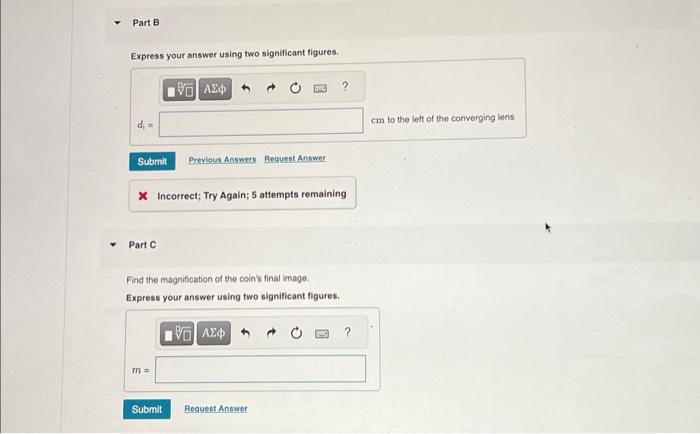Home / Expert Answers / Physics / a-converging-lens-of-focal-length-8-800-cm-is-19-0-cm-to-the-left-of-a-diverging-lens-of-focal-lengt-pa666

# (Solved): a converging lens of focal length 8.800 cm is 19.0 cm to the left of a diverging lens of focal lengt ...

a converging lens of focal length 8.800 cm is 19.0 cm to the left of a diverging lens of focal length -8.00 cm. a coin is placed 18.0 cm to the right of the diverging lens.Exoress vour answer using two significant figures. X Incorrect; Try Again; 5 attempts remaining Part C Find the magnification of the coin's final image. Express your answer using two significant figures.

We have an Answer from Expert